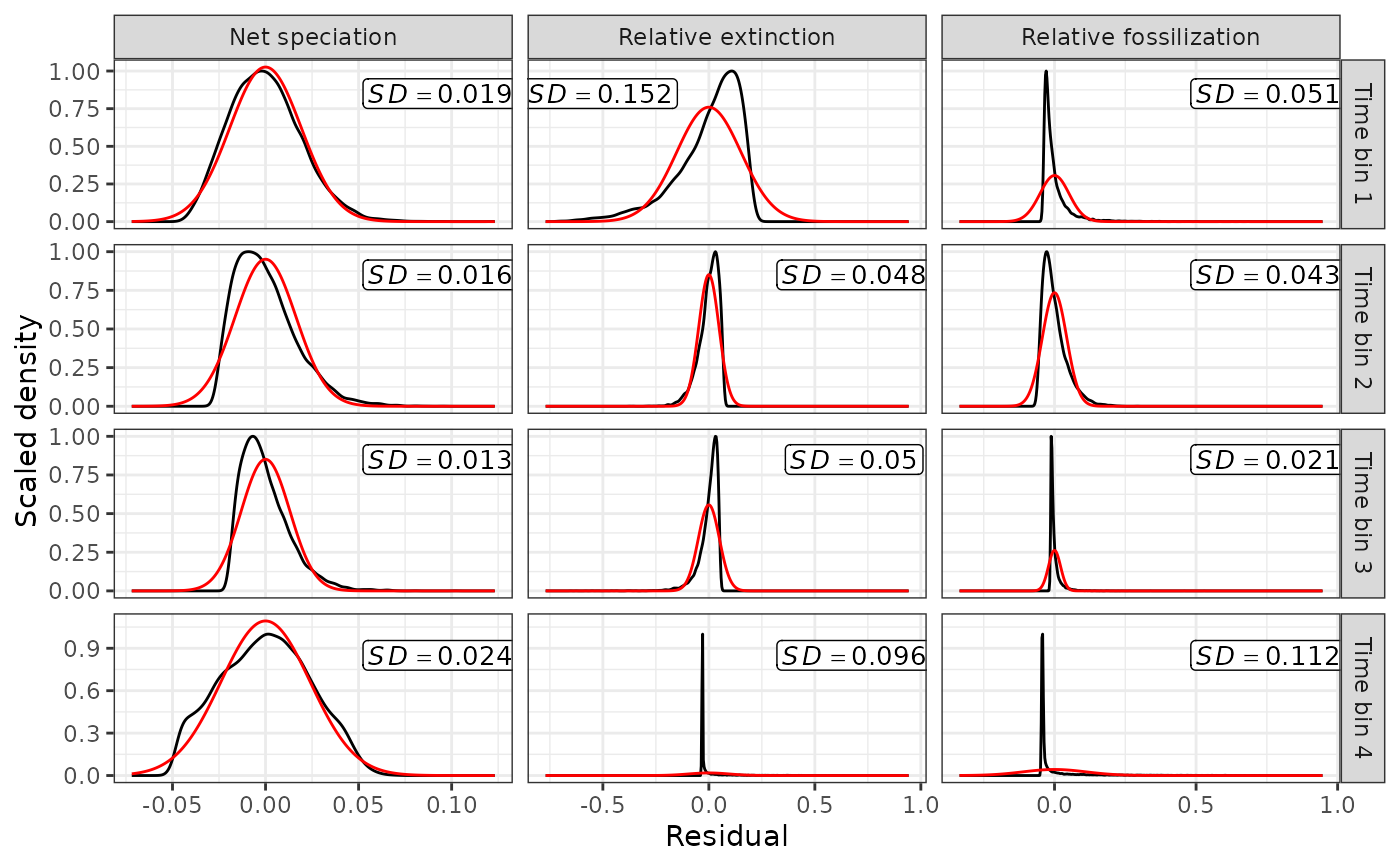Produces plots of the distributions of fossilized birth–death process (FBD) parameters to facilitate the assessment of the assumptions of normality within time bins and homogeneity of variance across time bins.

## Usage

FBD_normality_plot(posterior)

## Arguments

posterior

A data frame of posterior parameter estimates containing a single "Time_bin" column and one column for each FBD parameter value. Such data frame can be imported using combine_log followed by FBD_reshape.

## Details

The plots produced include density plots for each parameter within each time bin (residualized to have a mean of zero), scaled so that the top of the density is at a value of one (in black). Superimposed onto these densitys are the densities of a normal distribution with the same mean and variance (and scaled by the same amount) (in red). Deviations between the normal density in red and the density of the parameters in black indiciate deviations from normality. The standard deviation of each parameter is also displayed for each time bin to facilitate assessing homogenity of variance.

## Value

A ggplot object, which can be modified using ggplot2 functions.

vignette("fbd-params") for the use of this function as part of an analysis pipeline.

combine_log for producing a single data set of parameter posterior samples from individual parameter log files.

FBD_reshape for converting posterior parameter table from wide to long format.

FBD_tests1 for statistical tests of normality and homogeneity of variance.

FBD_tests2 for tests of differences in parameter means.

## Examples

# See vignette("fbd-params") for how to use this
# function as part of an analysis pipeline

data("posterior3p")

posterior3p_long <- FBD_reshape(posterior3p)

FBD_normality_plot(posterior3p_long)